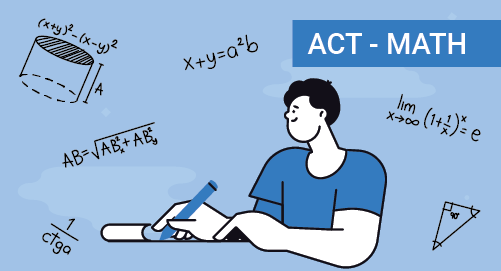#### Need Help?

Get in touch with usACT

# ACT Mathematics

Mathematics

ACT or the American Collee Testing Exam is a standardised test for the admission to bachelor course from IVY league colleges. ACT exam evaluates the student on four major ares like Maths, Science, language and writing areas. ACT assesses the students' performance in punctuation, grammar, sentence strucure, etc, in Maths, it covers the student's mathematical skills.

ACT (American College Testing) is a standardized test used for college admissions in the United States. It assesses a student's knowledge and skills in English, mathematics, reading, and science reasoning. The ACT test is administered by ACT, Inc. and is accepted by most colleges and universities in the US.

The ACT test consists of four main sections: English, Math, Reading, and Science. Additionally, there is an optional Writing section that some colleges may require or recommend. Here are some key details about each section:

English (45 minutes): This section measures your understanding of English grammar, punctuation, usage, sentence structure, and rhetorical skills. It includes multiple-choice questions and a passage with underlined portions where you need to identify errors or make improvements.

Math (60 minutes): The Math section evaluates your mathematical skills in areas such as algebra, geometry, trigonometry, and basic statistics. It includes multiple-choice and grid-in questions that require you to solve problems and apply mathematical concepts.

Reading (35 minutes): In the Reading section, you'll be presented with four passages from various subjects and asked questions to assess your comprehension, interpretation, and analysis skills. The passages may include prose fiction, humanities, social science, and natural science.

Science (35 minutes): The Science section measures your scientific reasoning abilities rather than specific content knowledge. You'll be given several sets of scientific data, charts, graphs, and experiments, and you'll need to analyse and interpret the information to answer questions.

Writing (optional, 40 minutes): The optional Writing section requires you to write an essay in response to a prompt. It assesses your ability to develop a clear argument, support it with relevant evidence, and showcase your writing skills. While the Writing section is not included in the composite score, some colleges may require it for admission.

## Test Pattern

S No Section Number of Questions Time Allotted
1 English 75 45 minutes
2 Math 60 60 minutes
4 Science 40 35 minutes
5 Writing* Essay Prompt 40 minutes

*The Writing section is optional and does not include a specific number of questions. It requires writing an essay in response to a prompt

## What you'll learn

• 1

Linear Equations in 1-variable and 2-variables

• 2

Graphical representation of Algebraic Expressions

• 3

Ratio, proportion, rate and percentage

• 4

Units, Measurement and unit conversion

• 5

Probability and Two-way Frequency Tables

• 6

• 7

Data collection and Representation

• 8

• 9

3D shapes and Solid Geometry

• 10

Lines, Angles, Triangles, Pythagorean Theorem and Trigonometric Ratios

• 11

Cicles, basic concepts of circles and Complex Numbers

## Skills you'll learnCreate and solve linear equations, and inequalities in 1 variable and 2 variablesRelation between Graphical representation and Algebraic representationSolve problems using ratios, proportions, rate, and percentageMeasurement of different quantities, their units and unit conversionUsing two-way frequency tables and calculate probabilityUse of relationship between two variables through scatterplot, linear, quadratic and exponential models to investigate the graphsDifferent methods of data collection, representing in tabular formats, calculating mean, median, range, etcWriting Polynomials and basic operations on polynomialsSimplifying and solving radical functions and rational functionsUnderstand congruency and similarity. Apply for lines, angles and trianglesUnderstand trigonometric ratios and use trigonometric functions to measure anglesSimplify Complex Numbers and Basic Operations of Complex Numbers